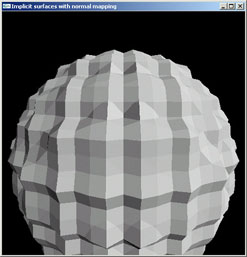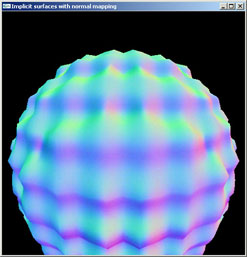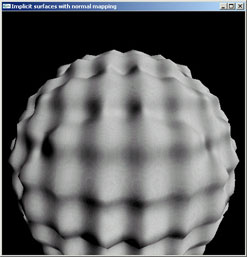Implicit Surface With Normal Map Extraction Using Marching Cubes

by
Mark Barry

CSC 570, Winter 2006

Motivation

A high resolution mesh generated from volume data or an implicit surface is generally very large and hard to work with.  Many small triangles are needed to capture the fine surface details.

Normal mapping a low resolution mesh is an efficient way to convey fine surface details while maintaining a simple underlying geometry.  Here are the traditional steps required to generate a low resolution mesh and extract a associated normal map:

• Generate a super high resolution mesh from volume data or an implicit surface using the Marching Cubes algorithm.  This may generate a mesh numbering in the millions of triangles.
• Simplify the mesh using some sort of mesh simplification algorithm such as Quadric Error Metrics.
• Load the high resolution and low resolution meshes into normal-map-generating software such as nVidia's Melody or ATI's NormalMapper.  These programs will extract the normals from the high resolution mesh and create a normal map for the low resolution mesh.

The motivation of this project is to short-cut the process but generating a low resolution mesh along with the corresponding normal map all in one step.  This cuts out the steps of generating and managing a cumbersome high resolution mesh, simplifying it, and then running through a normal map generator.  All these extra steps are time consuming and need to be done manually.

Goal

The goal of this project is to create a program that extracts a low resolution surface and the appropriate normal map from an implicit surface.  Then display the triangle mesh with applied normal map using OpenGL.

Algorithm

• Generate a triangle mesh from an implicit surface using the Marching Cubes algorithm.
• The marching cubes are relatively small and generate only a rough mesh conveying the basic shape of the surface.
• For each triangle in the generated mesh:
• Define a rectangle bounding the triangle as dimensions u x v.   Allocate a normal map of these dimensions for the triangle.
• Sample across the triangle in the u and v directions extracting the surface normals to create the normal map for that triangle.  Surface normals are calculated by evaluating the implicit surface's gradient at the current sampling (x,y,z) point on the triangle.
• Display the triangle mesh with applied normal maps using OpenGL.

Results

Using a simple sphere implicit surface is not interesting enough.  Instead, the following implicit equation makes for and interesting surface with finer detail:Where a = 0.6, b = 7.4, and c = 20Flat shaded surface Textured mapped surface with RGB values corresponding to XYZ components of the surface normal Normal mapped surface

The mesh contains 8,216 triangles.  As can be seen, the normal mapping visually smoothes the surface, correcting the normals, while still maintaining the same underlying geometry.  Generating the mesh, normal maps, and outputting the display take only a few seconds.